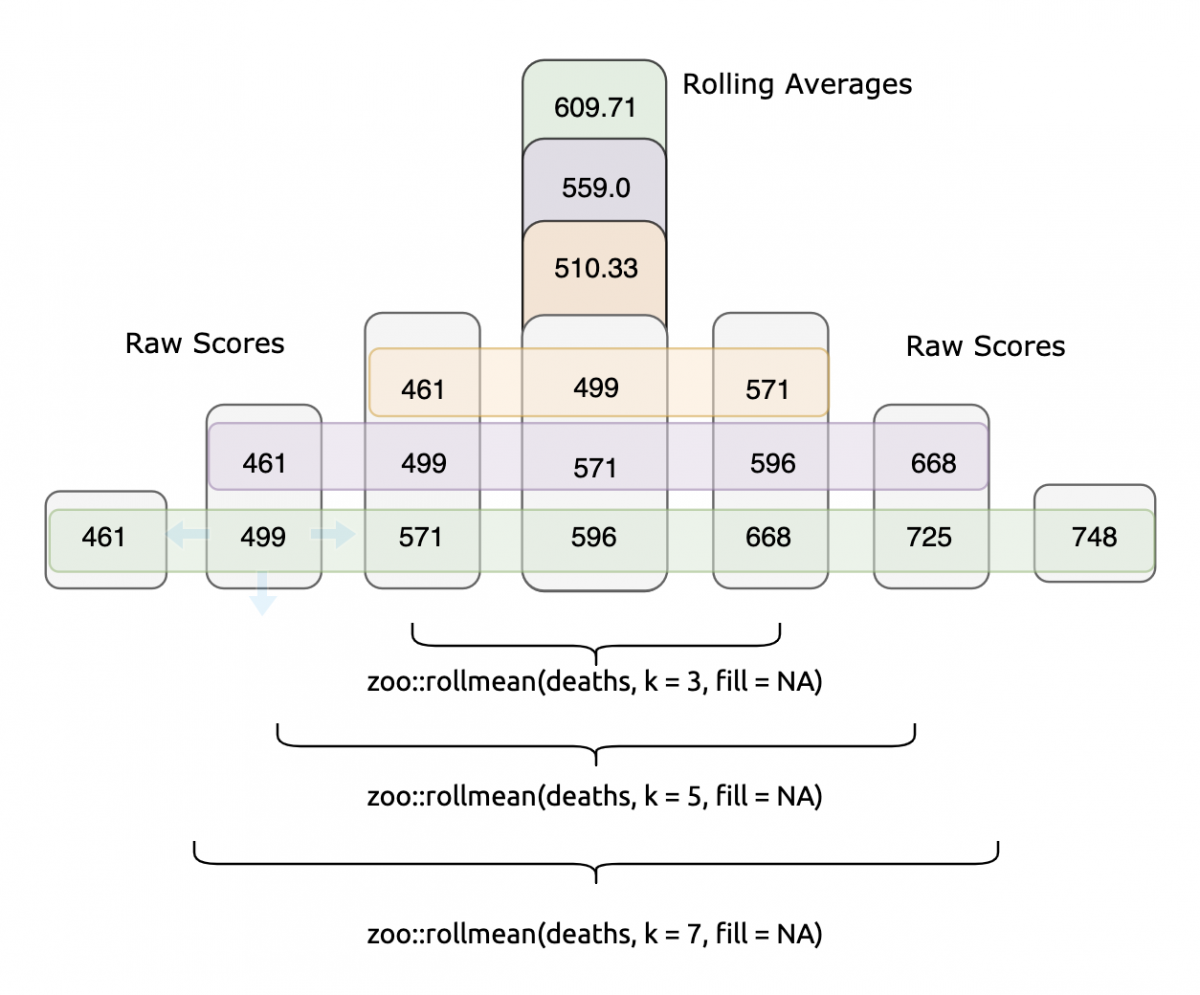# 16 Smoothing MethodsMoving-average forecast models use the average of the last $$k$$ observed values to forecast next period’s value. The number $$k$$ is called the span of the moving average. Larger values of $$k$$ result in a smoother model.

The forecast equation for the simple exponential smoothing model is a weighted average of last period’s observed value and last period’s forecasted value. The degree of smoothing is determined by the choice of a smoothing constant $$w$$ . Some practitioners restrict $$w$$ to the range of $$0\leq w \leq 1$$, but some software will allow for a range of $$0 \leq w &lt; 2$$.